# 5. Formulae

 DEFINITION: A formula performs calculations using numbers, addresses of cells and mathematical operators

Formulas are the bread and butter of spreadsheets. Without formulas, a spreadsheet would only contain a static never-changing set of numbers. Not exactly useful.

A formula takes a set of values, usually from other cells, and carries out some maths on them. The result is displayed in the same cell containing the formula.

Normally formulas are not visible when just viewing a spreadsheet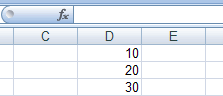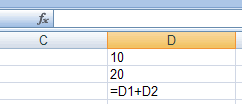The image on the left is what you would normally see in a spreadsheet. But if you set the spreadsheet software to make formulas visible, then you see the image on the right. Just how you make formulas visible depends on the package, so check with the help menu.

Formulas can also contain functions which are explained in the next page. Like this.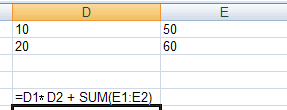The normal maths operators are combined with variable or cell references and / or functions. A formula can only calculate a single value within a single cell.

#### Mistakes in formulas

The picture above shows a formula that multiplies D1 by D2 and then adds the result to E1 + E2.

What happens to the formula if you then delete Row 1? You get this...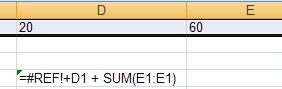A #REF error appears as the formula cannot work. The spreadsheet will no longer calculate the formula and it is up to you to sort out the problem.

With large spreadsheets containing tens of thousands of formulas, deleting a single cell location can result in thousands of #REF errors appearing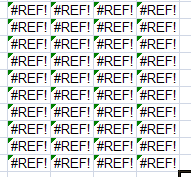Another way of making a mistake is to include a named variable that does not / no longer exists. Like this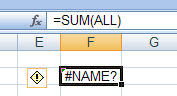There is no 'ALL' named variable in this spreadsheet so the #NAME message appears in the cell containing the formula.

So formulas are very powerful, but you do need to make sure that it can actually carry out the calculation sensibly.

challenge see if you can find out one extra fact on this topic that we haven't already told you